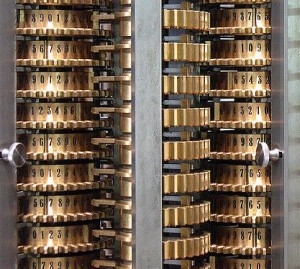Certain probabilists and statisticians like to repeat that the Metropolis-Hastings algorithm is one of the most important algorithms on earth, and drop the name of Diaconis to reinforce the statement. Well, beyond earth, this might be true in the whole universe, who knows?

Let us recall that the Metropolis-Hastings algorithm is a remarkable recipe to produce a Markov kernel which can be simulated and which admits a prescribed probability distribution, known up to a multiplicative constant, as its unique reversible invariant distribution. The idea behind the Metropolis-Hastings algorithm is very simple, and can be traced back to an article published in 1953 entitled Equation of State Calculations by Fast Computing Machines, written by Nicholas Metropolis, Arianna & Marshall Rosenbluth, and Augusta & Edward Teller, and to an article published in 1970 entitled Monte Carlo Sampling Methods Using Markov Chains and Their Applications, written by W. Keith Hastings. It mixes the idea of rejection sampling of Georges-Louis Leclerc Comte de Buffon and John von Neumann with the Markov chain concept. By the way, Edward Teller is among other things credited as being the mother of the US H-bomb together with the father Stanislaw Ulam (who was by the way fond of the Monte-Carlo method). Nowadays, the Metropolis-Hastings algorithm is available in many versions and is at the heart of the so-called Monte-Carlo-Markov-Chain (MCMC) numerical algorithms.

Yes the Metropolis-Hastings algorithm is a remarkable widely used non exact algorithm for stochastic simulation. However, the most important algorithms used in the world are probably more related to information/signal processing,  number crunching, linear algebra, and optimization, such as the Huffman coding algorithm, the Lempel-Ziv-Welch coding algorithm, the Hamming coding algorithm, the Reed-Muller coding algorithm, the Gallager coding algorithm, the Gram-Schmidt algorithm, the QR algorithm, the LU algorithms,  the Gauss elimination algorithm, the Euclidean algorithm, the Newton algorithm, the Simplex algorithm, the Hungarian algorithm, the Fast Fourier Transform algorithm, etc.

Hi Djalil,

to be precise, at least according to Bethe (http://en.wikipedia.org/wiki/History_of_the_Teller%E2%80%93Ulam_design), Teller was rather the mother of the H-bomb 😉 :

“For the sake of history, I think it is more precise to say that Ulam is the father, because he provided the seed, and Teller is the mother, because he remained with the child. As for me, I guess I am the midwife.”

Best,